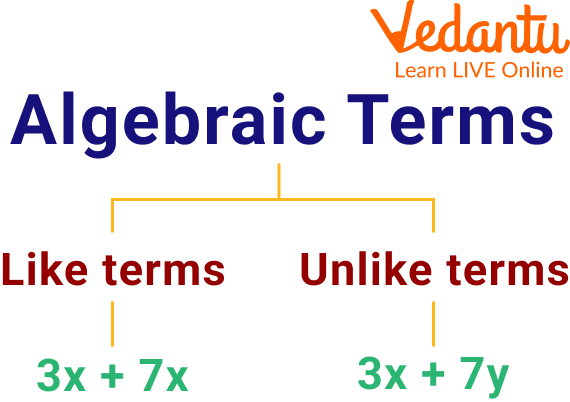Courses
Courses for Kids
Free study material
Free LIVE classes
More

# Subtraction of Unlike TermsLIVE
Join Vedantu’s FREE Mastercalss

## Introduction to the Subtraction of Unlike Terms

Have you ever thought about whether one can obtain a single result on the subtraction of two unlike terms or not? Subtraction is the process of removing a part of a given set of similar things to obtain the result. There will be no result from subtracting two non-similar things. Thus, it can be said that, unlike terms, never produce a single result when subtracted. These give a result as the difference between two, unlike terms. Let's read the article to learn further about the topic.Algebraic Terms

## Define Unlike Terms

Unlike terms are algebraic terms that have different variables. These can not be put up to the identical power of a number. For example, 7xy + 9x - z constitutes an, unlike term.

## Subtraction of Two Positive Unlike Terms

To calculate the difference between two positive unlike terms, let us consider two positive numbers, say m and n, then subtract n from m, we must connect both the terms with a subtraction sign and express the result as m - n.

## Subtraction of Positive and Negative Unlike Terms

If we take two numbers, one positive, say m and one negative, say -n. Then, subtract -n from m, by using a subtraction sign i.e. $[m-(-n)]$. The outcome thus obtained to discover the difference can be expressed in the form of m + n.

## Subtraction of Negative and Positive Unlike Terms

Let us consider two numbers, one negative, say $-\mathrm{m}$, and the other positive, say $\mathrm{n}$. If we want to remove n from -m, we must connect them by using the subtraction symbol i.e. $[(-m)-n]$. The obtained result of the difference between two opposite sign terms can be expressed in the form of (-m-n).

## Subtraction of Negative and Negative Unlike Terms

Assuming two negative numbers, say $-\mathrm{n}$ and $-\mathrm{m}$. Let us take the difference of $-\mathrm{n}$ from $-\mathrm{m}$, by using the subtraction symbol i.e. $[(-m)-(-n)]$. Here, the result of the difference between two negative numbers can be expressed in the form of -m+n.

## Solved Examples

1. Subtract 7z from 4y.

We need to subtract the given two, unlike both positive terms. Thus applying the result m-n, where m = 4y and n = 7z.

4y-7z is the required answer obtained by subtracting two positives, unlike terms.

Q 2. Find the difference between -4 and -7mn

We need to find the difference between the given two unlike terms, which are both negative. Thus applying the result $-m+n$, where $m=4$ and $-n=7 \mathrm{mn}$.

$-4+7 \mathrm{mn}$ is the required answer obtained by subtracting two negatives, unlike terms.

Q 3. Subtract - 2b from 10a.

Ans: We need to subtract the given two, unlike terms, out of which one is positive while the other is negative. Thus applying the result m + n, where m = 10a and -n = 2b.

$10 a+2 b$ is the required answer obtained by subtracting one positive and one negative, unlike a term.

## Practice Problems

Q 1. Find the difference between $11 \mathrm{pq}$ and $9 \mathrm{p}$.

Ans: $11 p q-9 p$

Q 2. Calculate the difference between $-9 p^2$ and $27 p$.

Ans: $\left(-9 p^2-27 p\right)$

Q 3. How much is $7 p+5 q$ greater than $3 q$?

Ans: $7 p+2 q$

Q 4. Find the difference between $13 x y$ and $-4 z$

Ans: $13 x y+4 z$

## Summary

Subtraction is one of the most basic topics of mathematics which the students must learn to master the calculations. It serves as the foundation for various topics including data handling, mensuration, etc. Some children find it difficult to learn this topic due to the unavailability of a better understanding of the concepts. This article provides you with all the details regarding the subtraction of unlike terms, the subtraction of two positive, one positive, and other negative unlike terms, and the subtraction of two negative unlike terms. Hoping you learned and enjoyed reading it.

Last updated date: 29th Sep 2023
Total views: 95.4k
Views today: 1.95k

## FAQs on Subtraction of Unlike Terms

1. Is it possible to subtract two like terms to produce a single result?

Yes, two like terms can be subtracted to produce a single result. For example: let us consider two like terms, say 4m and 8m. Their difference is given by subtracting 4m from 8m i.e. $8m - 4m = 4m$. The result is 4m, which is a single term.

2. Does the addition of unlike terms possibly give the same result?

No, the addition of unlike terms is not possible as addition and subtraction are similar procedures, the only difference is that one is used to sum up all the quantities while the other is used to remove some of the quantities.  For example, $3xy + z$ does not add up to give a single result.

3. Define like terms.

Like terms are defined as algebraic terms that contain the same variables. For example, $7xy + 9xy$. All the arithmetic operations can be performed on like terms.